# Electric currents Electromagnetism Microworld Macroworld Lecture 9 Electric

• Slides: 67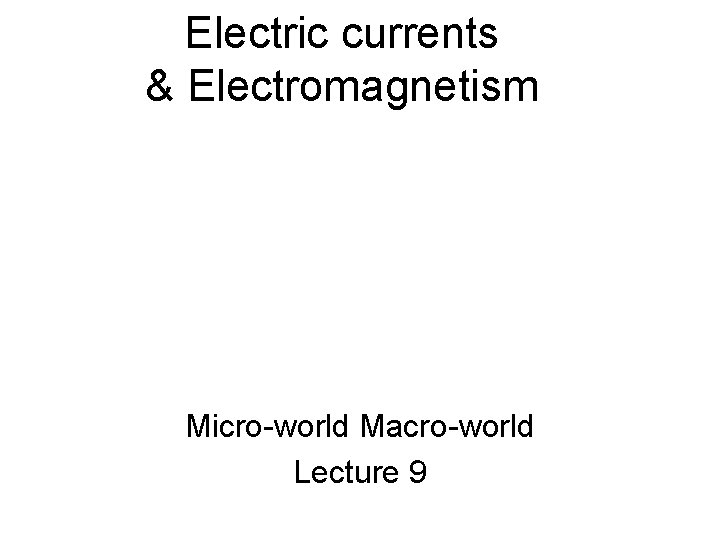Electric currents & Electromagnetism Micro-world Macro-world Lecture 9Electric currents (M oti on of ele c tri cc ha rge s) Micro-world Macro-world Lecture 9Alessandro Volta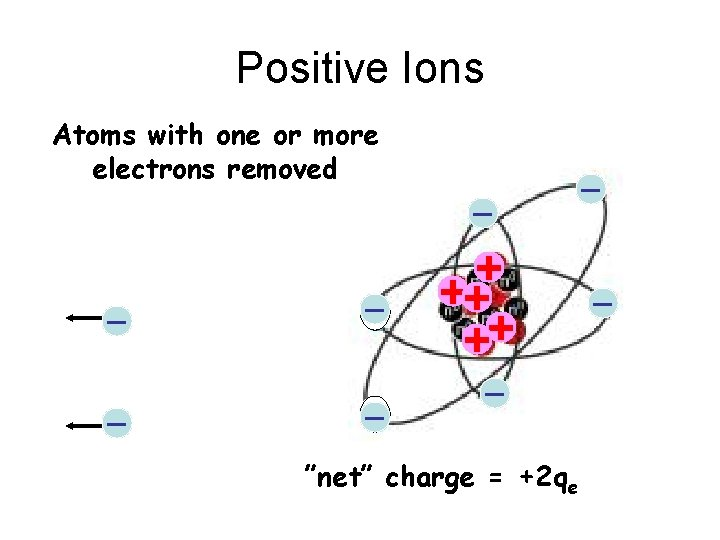Positive Ions Atoms with one or more electrons removed _ _ _ + ++ ++ _ ”net” charge = +2 qe _ _Battery C ++ -- + ++ + Zn Zn++ Zn - - Zn++ Zn Zn++ acid Zn Zn -Zn -“Voltage” Cathode Anode F = 2 qe. E + + + ++ + W = Fd E Zn++ F -- ” e g “V d W = 2 qe. Ed W 0 = 2 qe. E 0 d a t l o W 0 =E 0 d 2 qe VEnergy gaine d by the cha rge W = Fd =Q E 0 d = QV Q + + + ++ + Anode F=QE 0 Zn++ d Q F=QE 0 -- Cathode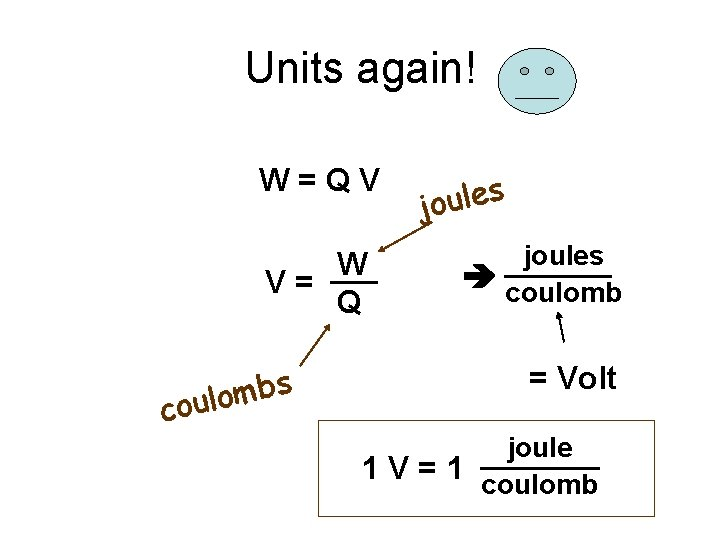Units again! W=QV W V= Q s b m coulo s e l u o j joules coulomb = Volt joule 1 V = 1 coulombContinuous charge flow = “electric current” Electrical “c onductor” co nnected between ano de & cathod e + + + ++ + Anode Q Q Zn++ -- Cathodeelectric current Q I= t + + + ++ + Anode Q Coulombs Units: second =Amperes Q Zn++ -- CathodeThe conductor can be a piece of wire Q I= t + + + ++ + Anode + Zn++ + -- Cathode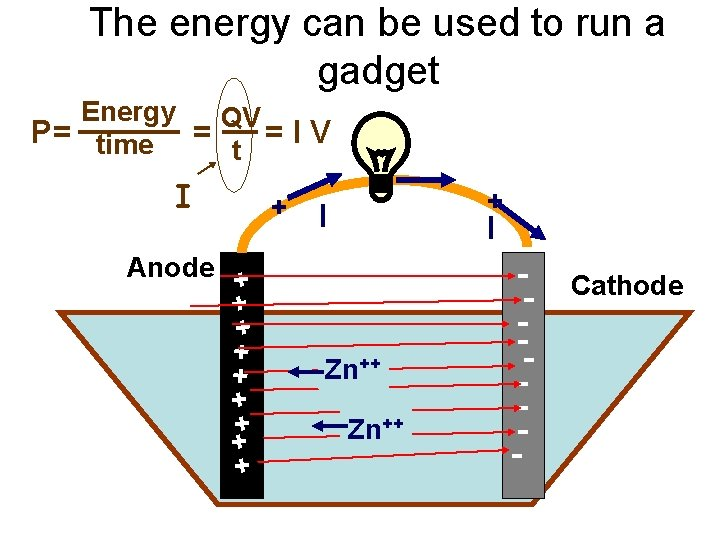The energy can be used to run a gadget Energy QV P= time = =IV t I + + + ++ + Anode + I + + I Zn++ -- Cathode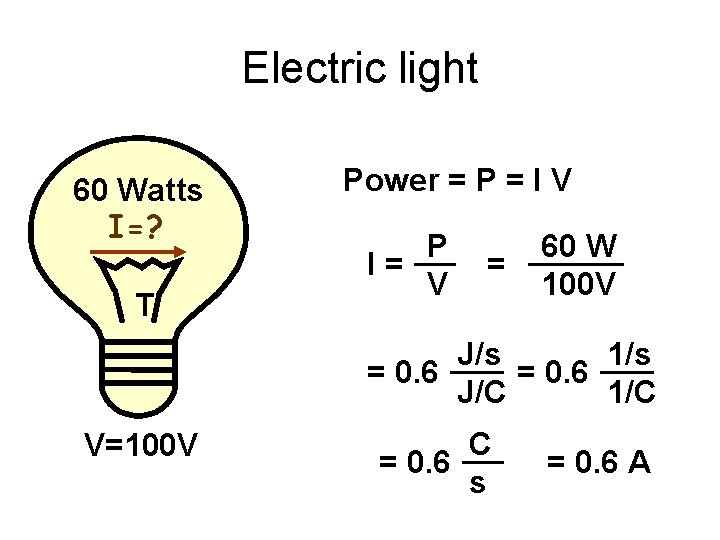Electric light 60 Watts I=? T Power = P = I V P I= V = 60 W 100 V J/s 1/s = 0. 6 J/C 1/C V=100 V C = 0. 6 s = 0. 6 AGeneral circuit I + Appliance + - 12 V I Energy source (device that separates + & - charge)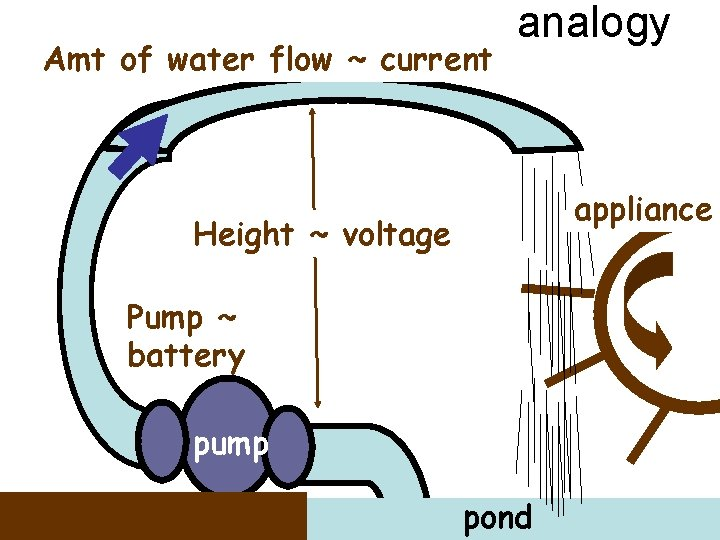Amt of water flow ~ current analogy appliance Height ~ voltage Pump ~ battery pump pondVoltas’ 1 st batteries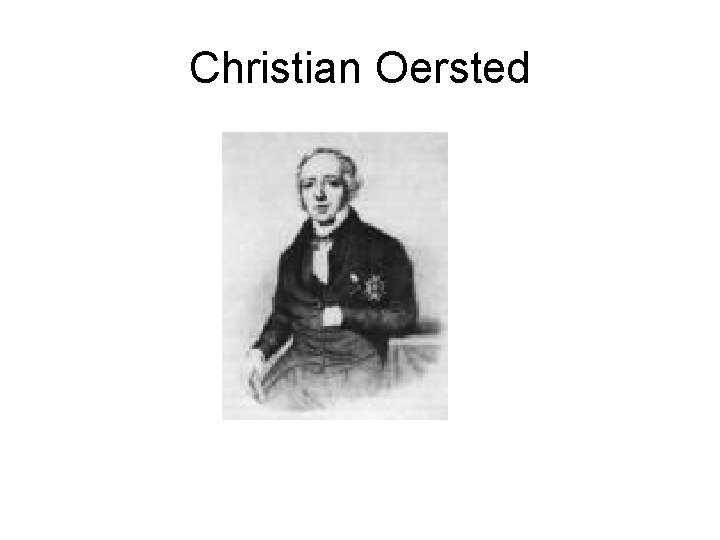Christian Oersted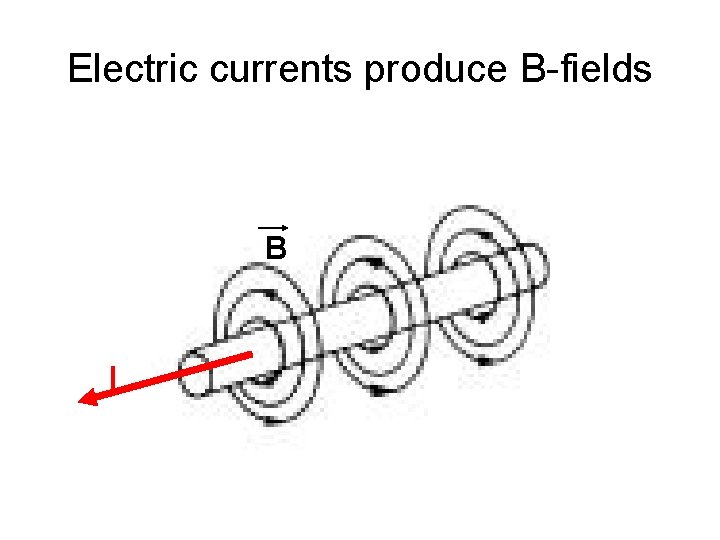Electric currents produce B-fields B I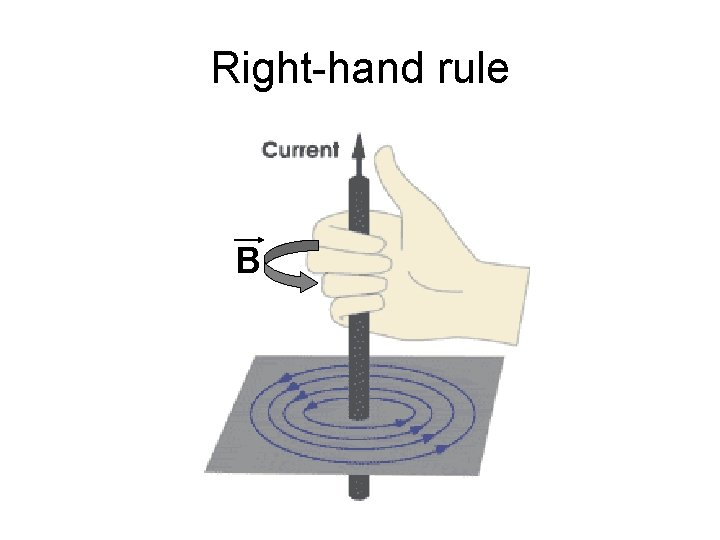Right-hand rule B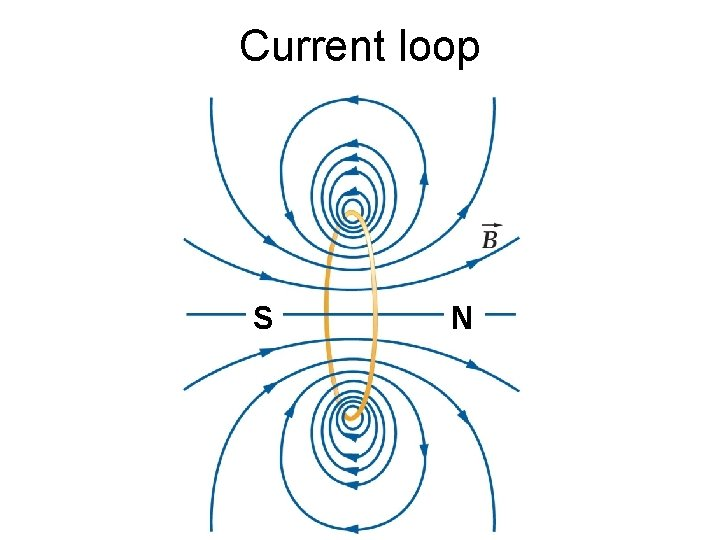Current loop S N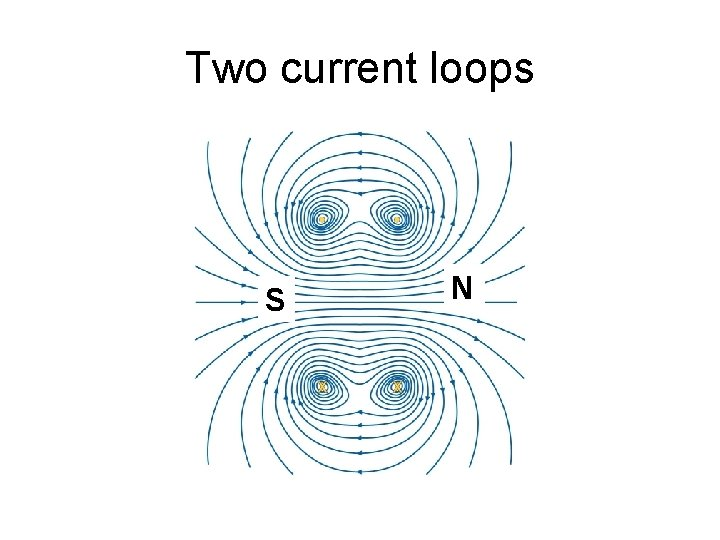Two current loops S NEven more loops S N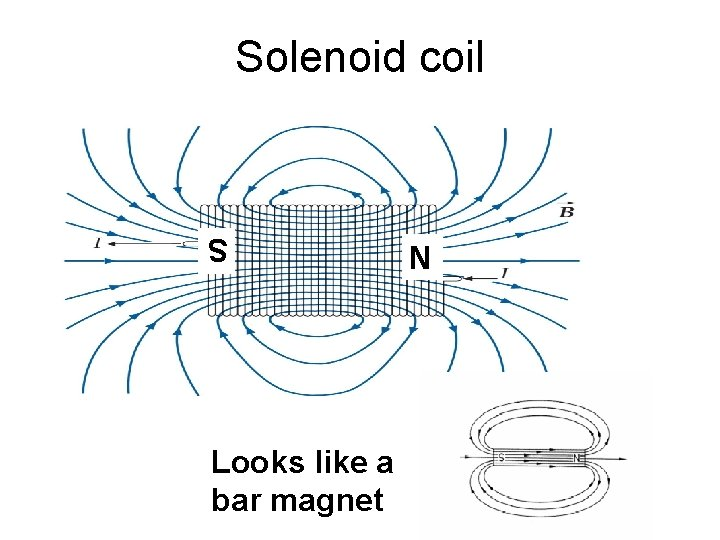Solenoid coil S Looks like a bar magnet NAtomic magnetism B + - I Some atoms are little magnets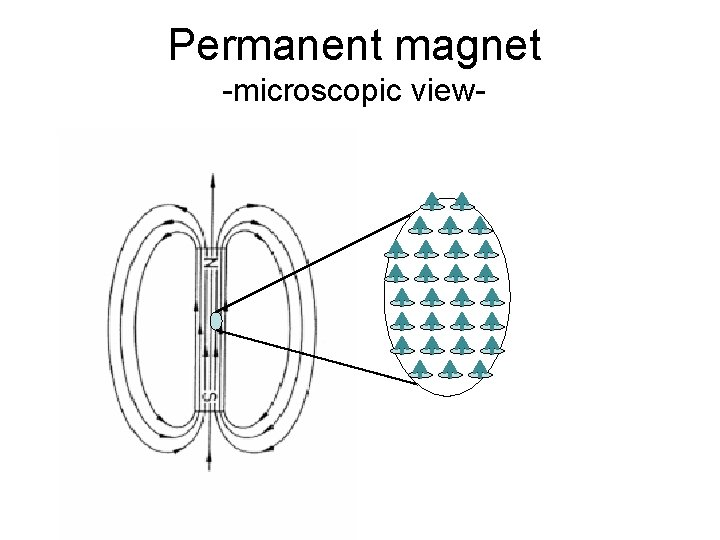Permanent magnet -microscopic view-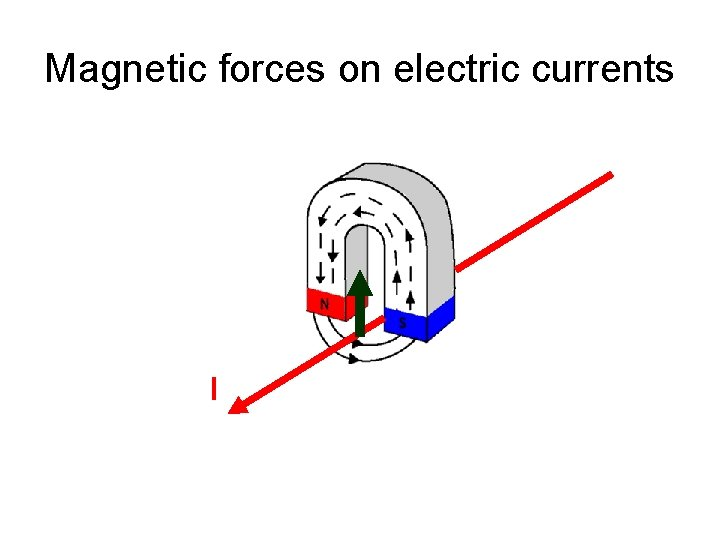Magnetic forces on electric currents I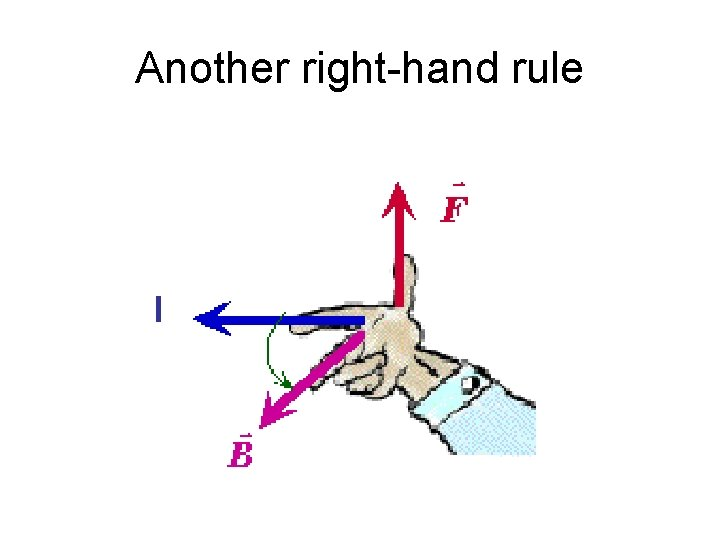Another right-hand rule IForces on two parallel wires I I B Current in same direction: wires attract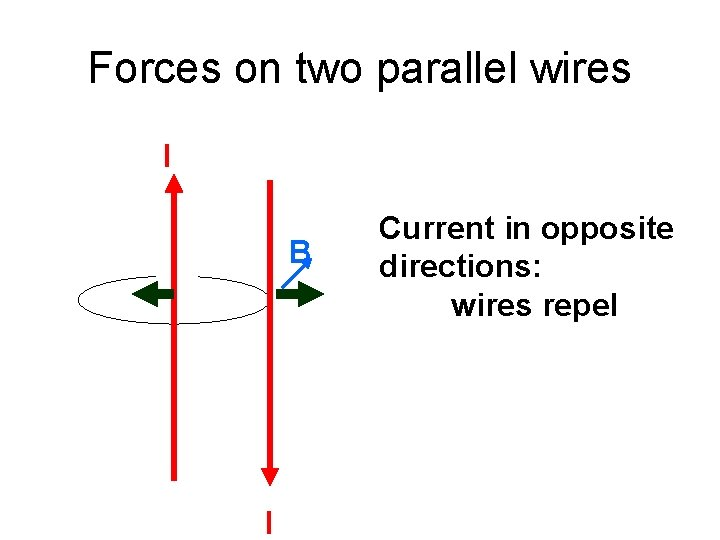Forces on two parallel wires I B I Current in opposite directions: wires repel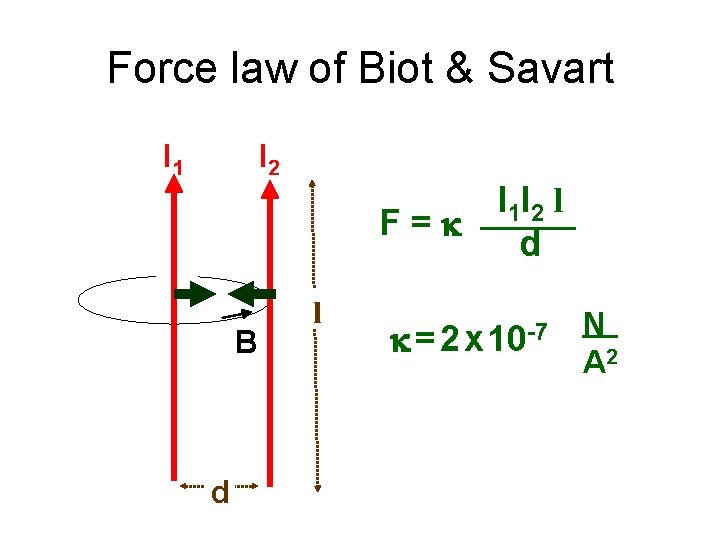Force law of Biot & Savart I 1 I 2 I 1 I 2 l F=k d B d l k = 2 x 10 -7 N A 2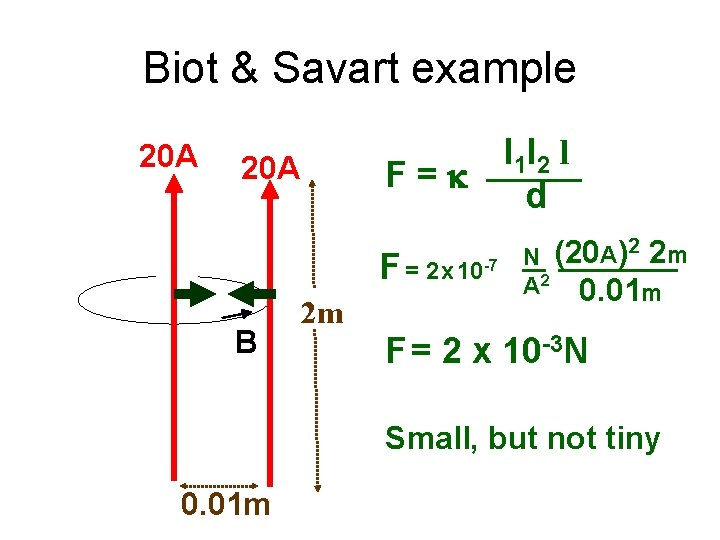Biot & Savart example 20 A I 1 I 2 l F=k d 20 A F = 2 x 10 -7 B 2 m N (20 A)2 2 m A 2 0. 01 m F = 2 x 10 -3 N Small, but not tiny 0. 01 mElectric motor F I I B FElectric motor B I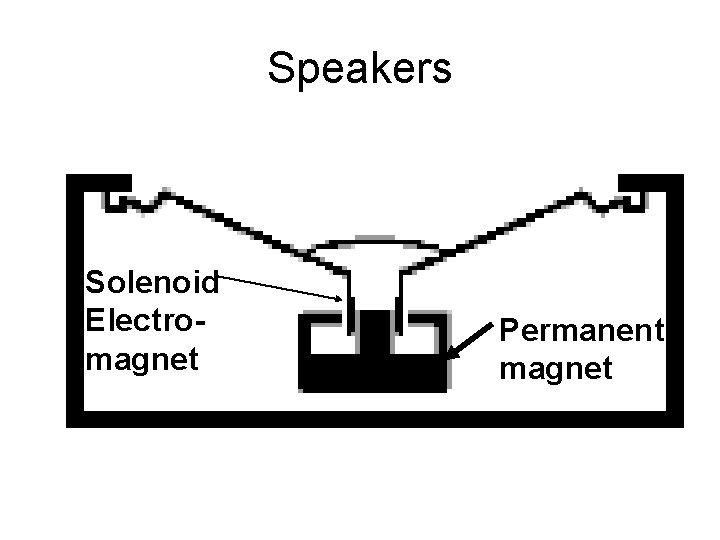Speakers Solenoid Electromagnet Permanent magnetLorentz force B v F +q if v B: i=qv F = i. B = qv. B direction by the right-hand ruleMoving a Conductor in a B-field separates + & - charges I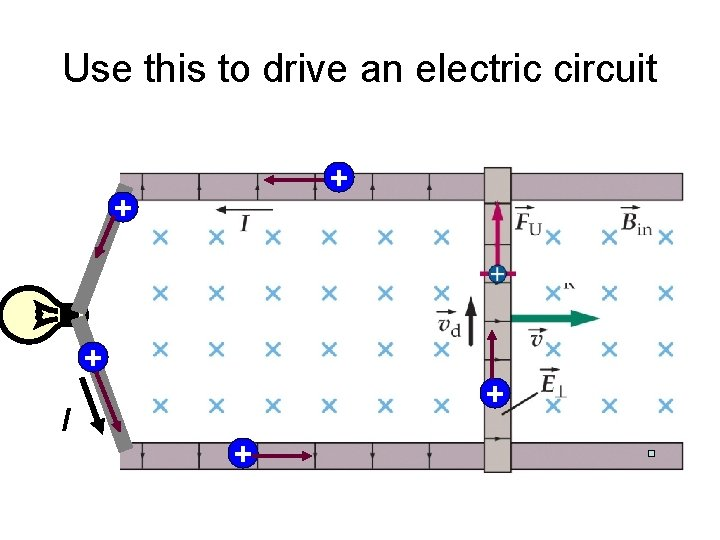Use this to drive an electric circuit + + + I + +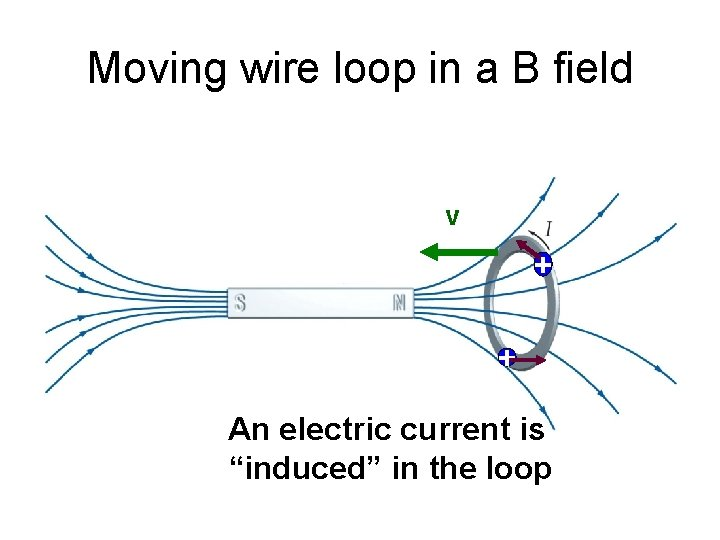Moving wire loop in a B field v + + An electric current is “induced” in the loopEither the magnet or the loop can move v + + an electric current is “induced” in the loopMagnetic flux (F) thru a loop F = BA┴Flux thru a coil of N loops F = N BA┴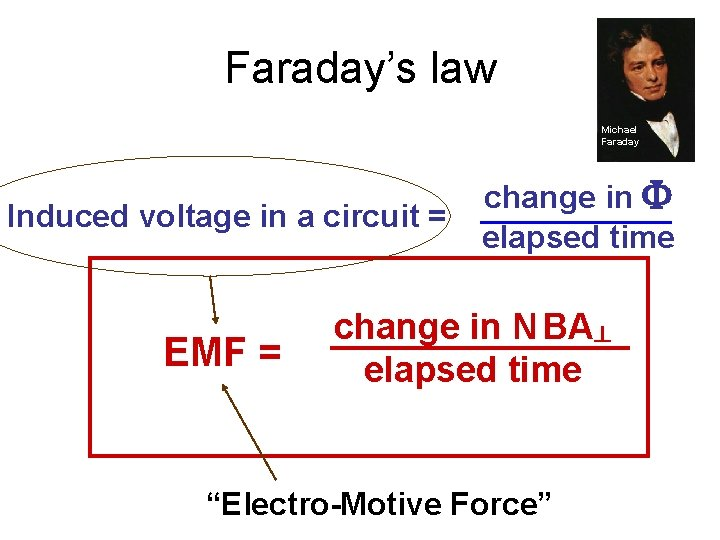Faraday’s law Michael Faraday Induced voltage in a circuit = EMF = change in F elapsed time change in N BA┴ elapsed time “Electro-Motive Force”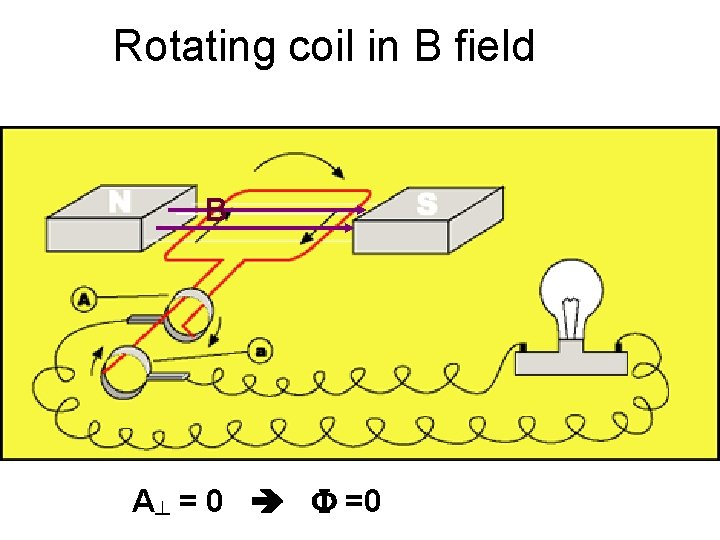Rotating coil in B field B A┴ = 0 F =0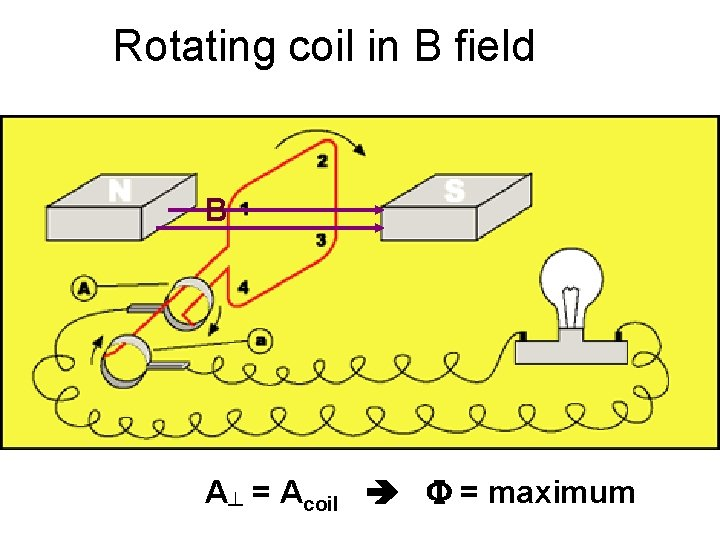Rotating coil in B field B A┴ = Acoil F = maximumRotating coil in B field B A┴ = 0 (again) F = 0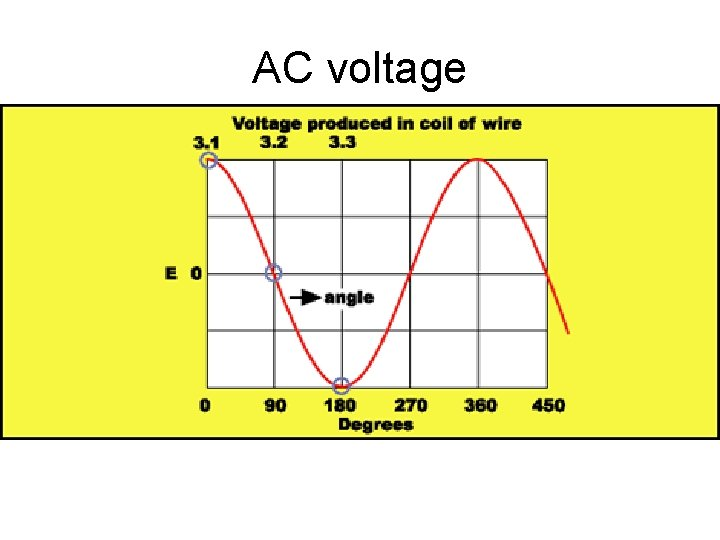AC voltage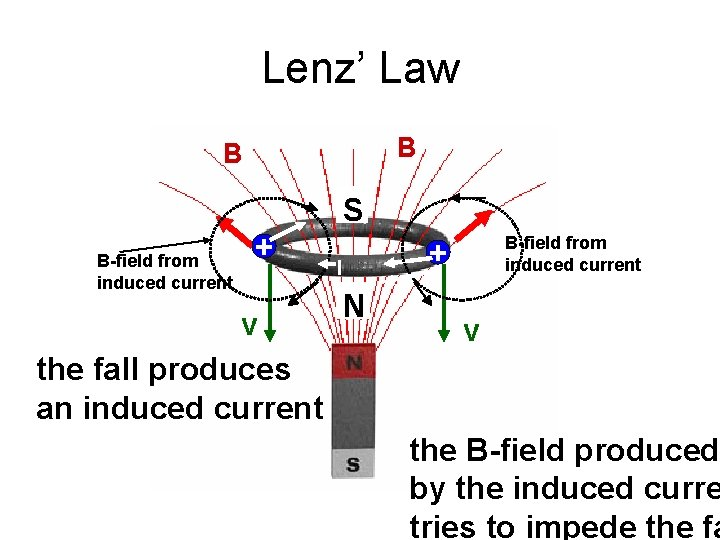Lenz’ Law B B S B-field from induced current + v the fall produces an induced current B-field from induced current + I N v the B-field produced by the induced curre tries to impede the fa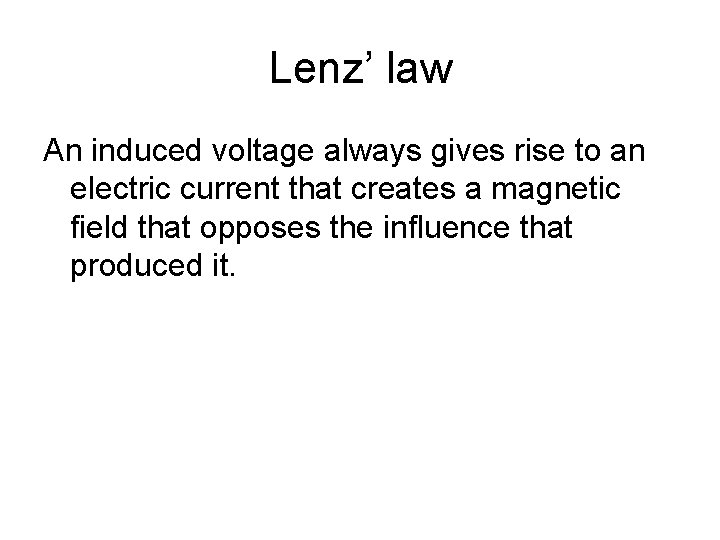Lenz’ law An induced voltage always gives rise to an electric current that creates a magnetic field that opposes the influence that produced it.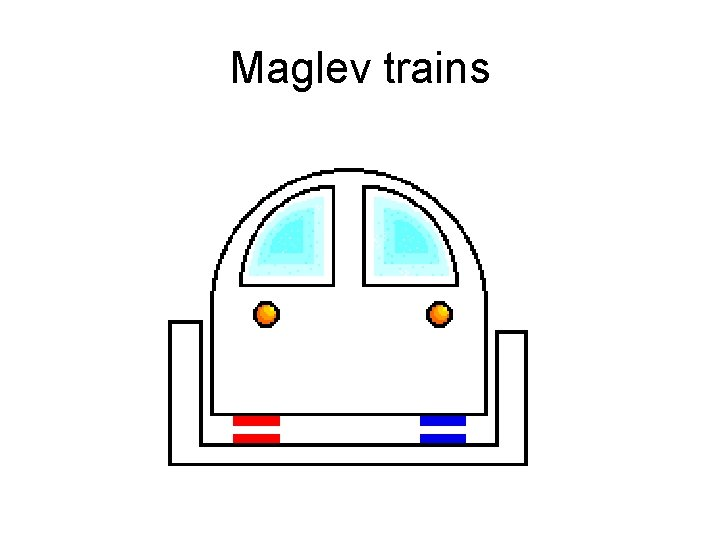Maglev trains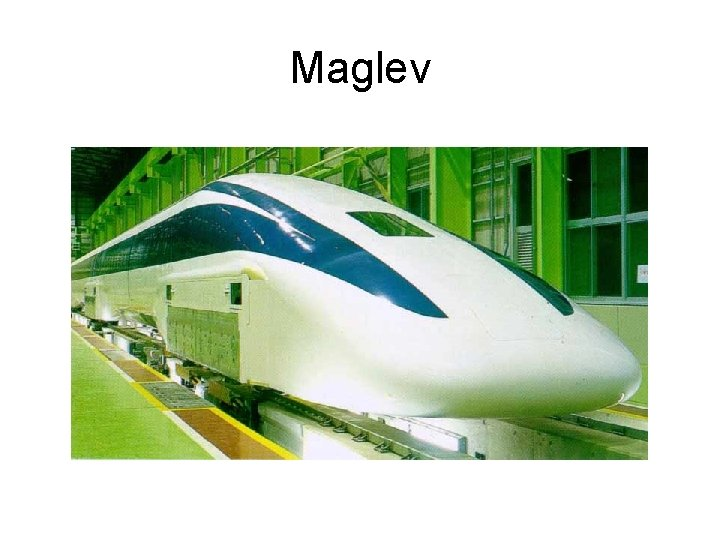MaglevMaxwell’s Equations James Clerk Maxwell “…and then there was light. ”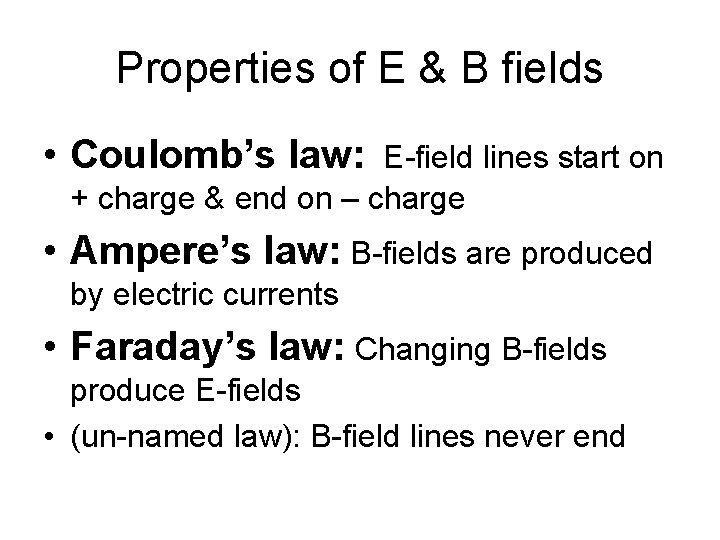Properties of E & B fields • Coulomb’s law: E-field lines start on + charge & end on – charge • Ampere’s law: B-fields are produced by electric currents • Faraday’s law: Changing B-fields produce E-fields • (un-named law): B-field lines never endIn equation form: E-field lines start on +charges & end on - charges B-field lines never end E-fields are produced by changing B fields B-fields are produced by electric currentsMaxwell The previous equations, as written, are mathematically inconsistent with the conservation of electric charge. He found he could fix this by adding one more term: B-fields are produced by changing E-fields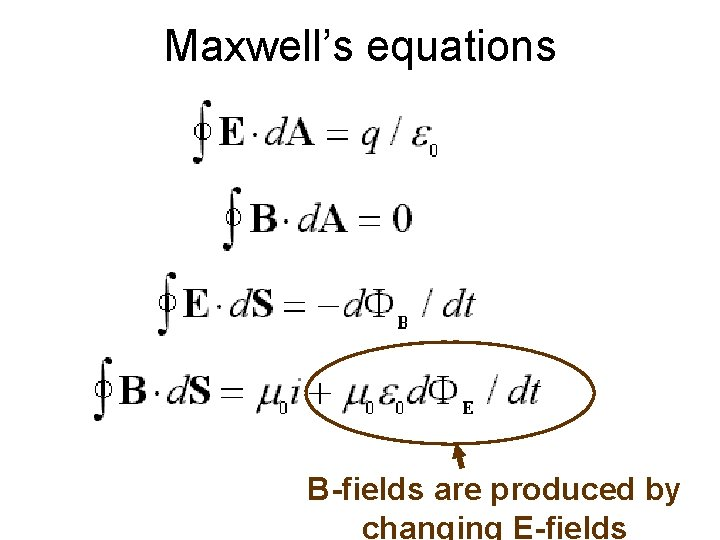Maxwell’s equations B-fields are produced by changing E-fieldsFields from an electric charge E x E Is the change in E instantaneous? Does it occur only after some time? + M. E. s can tell us? +fun in the bathtub Water level will increase but not instantaneously 1 st waves will propagate from her entrance point to the edge of the tubAccording to Maxwell’s eqs: E x E The change in E is not instantaneous 1 st waves made of Efields & B-fields propagate thru space. + +Wave solutions to Maxwell’s Eqs: Wave speed = = = electric force k = 9. 0 x 109 Nm 2/C 2 2 x 10 -7 N/A 2 9 x 109+7(m 2/C 2)x. A 2 3 x 108 m/s k ”strength” of magnetic force ! ! ht g i l f 16 m 2/s 2 e p S q 1 q 2 k r 2 Fc = 2 x 9 x 109 Nm 2/C 2 = 9 x 10 = k ”strength” of 2 k k o d e FM = k k=2 x I 1 I 2 l d 10 -7 N A 2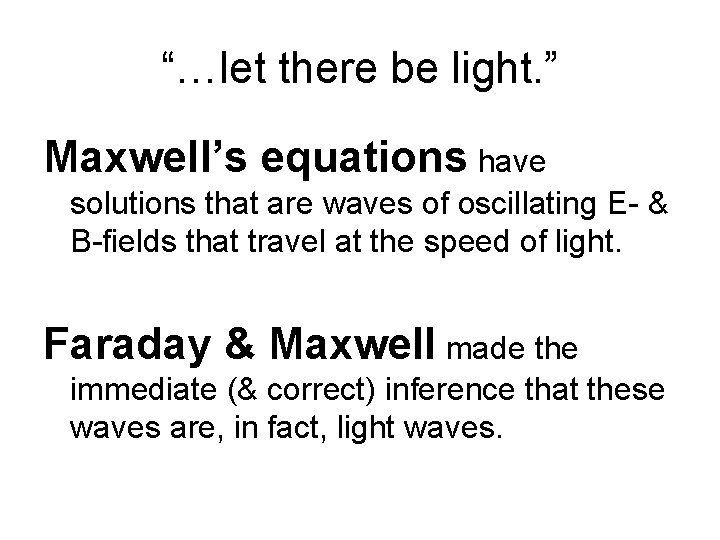“…let there be light. ” Maxwell’s equations have solutions that are waves of oscillating E- & B-fields that travel at the speed of light. Faraday & Maxwell made the immediate (& correct) inference that these waves are, in fact, light waves.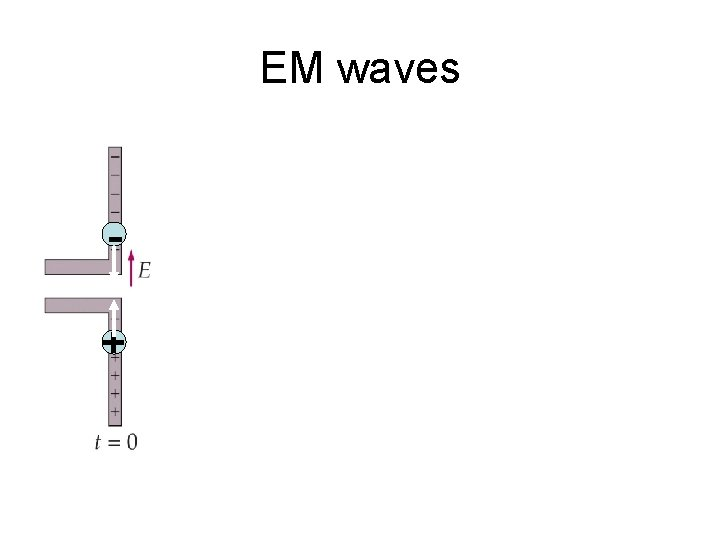EM waves + + + -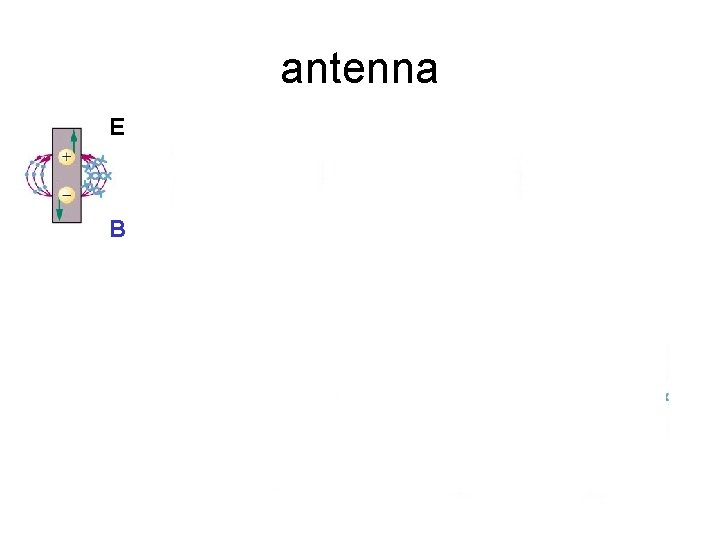antenna E B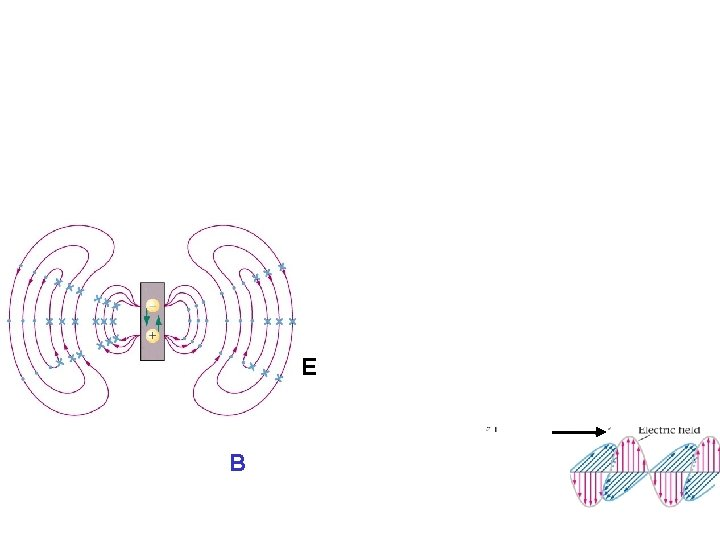antenna E BLight wave B-field + E-field wave velocityLight wave animation E Bl freq (c/l) 0. 75 x 10 -6 m 0. 55 x 10 -6 m 0. 4 x 10 -6 m 4. 0 x 1014 Hz 5. 5 x 1014 Hz 7. 5 x 1014 Hz Visible light: Red Green Violet Infrared Ultraviolet X-rays grays TV/FM AM radio waves micro waves Electro-magnetic “spectrum”